The “forecastHybrid” package provides functions to build composite models using multiple individual component models from the “forecast” package. These `hybridModel` objects can then be manipulated with many of the familiar functions from the “forecast” and “stats” packages including `forecast()`, `plot()`, `accuracy()`, `residuals()`, and `fitted()`.

# Installation

The stable release of the package is hosted on CRAN and can be installed as usual.

``install.packages("forecastHybrid")``

The latest development version can be installed using the “devtools” package.

``devtools::install_github("ellisp/forecastHybrid/pkg")``

Version updates to CRAN will be published frequently after new features are implemented, so the development version is not recommended unless you plan to modify the code.

# Basic usage

``library(forecastHybrid)``

## Quick start

If you don’t have time to read the whole guide and want to get started immediately with sane default settings to forecast the `USAccDeaths` timeseries, run the following:

``quickModel <- hybridModel(USAccDeaths)``
``## Fitting the auto.arima model``
``## Fitting the ets model``
``## Fitting the thetam model``
``## Fitting the nnetar model``
``## Fitting the stlm model``
``## Fitting the tbats model``
``forecast(quickModel)``
``````##          Point Forecast    Lo 80     Hi 80    Lo 95     Hi 95
## Jan 1979       8338.853 7921.826  8920.446 7658.078  9172.112
## Feb 1979       7510.228 6715.340  8035.616 6471.558  8270.451
## Mar 1979       8239.845 7201.565  8886.679 6885.514  9146.115
## Apr 1979       8530.068 7579.393  9194.629 7329.759  9500.477
## May 1979       9335.495 8158.939 10112.349 7870.987 10442.376
## Jun 1979       9761.422 8480.119 10525.745 8239.026 10878.297
## Jul 1979      10671.559 9222.271 11613.448 8866.034 11987.171
## Aug 1979      10005.787 9084.828 10830.331 8798.198 11224.086
## Sep 1979       9009.997 8281.314  9944.791 7892.801 10357.609
## Oct 1979       9244.044 8263.554 10198.510 7979.809 10629.548
## Nov 1979       8780.154 8027.617  9732.246 7589.183 10180.765
## Dec 1979       9093.339 8255.912 10255.628 7816.759 10720.971
## Jan 1980       8361.404 7485.300  9496.297 7033.420 10011.749
## Feb 1980       7567.098 6687.306  8749.398 6141.503  9295.201
## Mar 1980       8258.481 7024.581  9586.522 6623.713 10161.075
## Apr 1980       8544.003 7363.330  9940.466 6966.957 10542.396
## May 1980       9330.649 7816.676 10861.976 7468.391 11490.092
## Jun 1980       9748.961 8163.219 11280.306 7783.650 11933.559
## Jul 1980      10620.025 8624.658 12373.743 8192.718 13051.201
## Aug 1980       9975.804 8685.104 11596.927 8248.308 12297.755
## Sep 1980       8995.063 7949.455 10718.099 7261.423 11441.542
## Oct 1980       9234.034 7976.578 10978.824 7417.369 11724.196
## Nov 1980       8783.644 7623.215 10519.779 6856.540 11286.453
## Dec 1980       9088.871 7951.715 11050.531 7288.262 11837.932``````
``plot(forecast(quickModel), main = "Forecast from auto.arima, ets, thetam, nnetar, stlm, and tbats model")``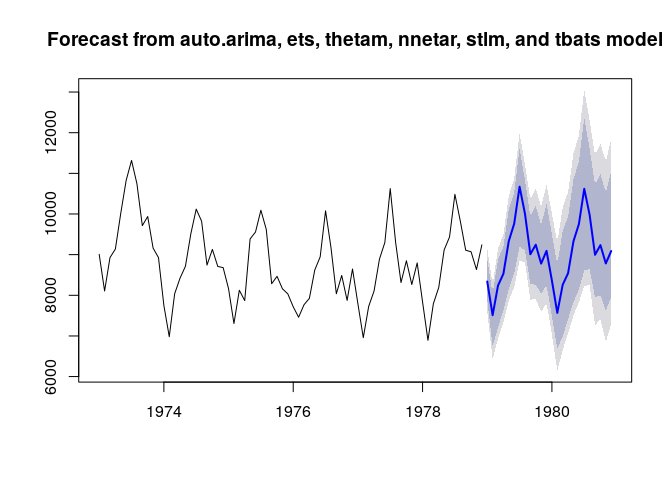## Fitting a model

The workhorse function of the package is `hybridModel()`, a function that combines several component models from the “forecast” package. At a minimum, the user must supply a `ts` or `numeric` vector for `y`. In this case, the ensemble will include all six component models: `auto.arima()`, `ets()`, `thetam()`, `nnetar()`, `stlm()`, and `tbats()`. To instead use only a subset of these models, pass a character string to the `models` argument with the first letter of each model to include. For example, to build an ensemble model on a simulated dataset with `auto.arima()`, `ets()`, and `tbats()` components, run

``````# Build a hybrid forecast on a simulated dataset using auto.arima, ets, and tbats models.
# Each model is given equal weight
set.seed(12345)
series <- ts(rnorm(18), f = 2)
hm1 <- hybridModel(y = series, models = "aet", weights = "equal")``````
``## Fitting the auto.arima model``
``## Fitting the ets model``
``## Fitting the tbats model``

The individual component models are stored inside the `hybridModel` objects and can viewed in their respective slots, and all the regular methods from the “forecast” package could be applied to these individual component models.

``````# View the individual models
hm1\$auto.arima``````
``````## Series: y
## ARIMA(0,0,0) with zero mean
##
## sigma^2 estimated as 0.6659:  log likelihood=-21.88
## AIC=45.76   AICc=46.01   BIC=46.65``````
``````# See forecasts from the auto.arima model
plot(forecast(hm1\$auto.arima))``````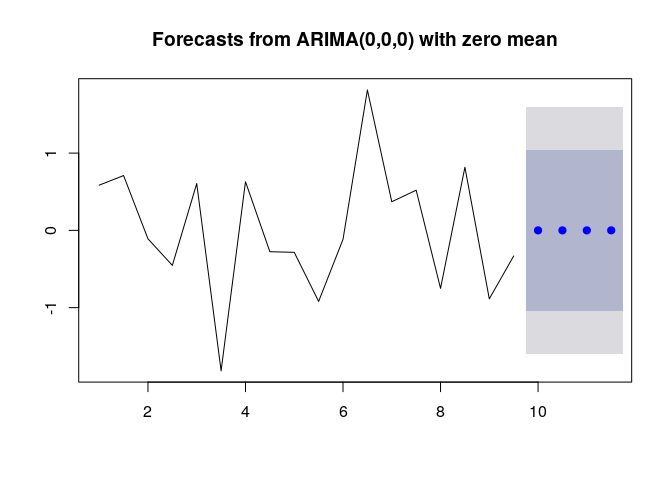### Model diagnostics

The `hybridModel()` function produces an S3 object of class `forecastHybrid`.

``class(hm1) ``
``##  "hybridModel"``
``is.hybridModel(hm1)``
``##  TRUE``

The `print()` and `summary()` methods print information about the ensemble model including the weights assigned to each individual component model.

``print(hm1) ``
``````## Hybrid forecast model comprised of the following models: auto.arima, ets, tbats
## ############
## auto.arima with weight 0.333
## ############
## ets with weight 0.333
## ############
## tbats with weight 0.333``````
``summary(hm1)``
``````##            Length Class          Mode
## auto.arima 18     forecast_ARIMA list
## ets        19     ets            list
## tbats      21     bats           list
## weights     3     -none-         numeric
## frequency   1     -none-         numeric
## x          18     ts             numeric
## xreg        1     -none-         list
## models      3     -none-         character
## fitted     18     ts             numeric
## residuals  18     ts             numeric``````

Two types of plots can be created for the created ensemble model: either a plot showing the actual and fitted value of each component model on the data or individual plots of the component models as created by their regular S3 `plot()` methods. Note that a `plot()` method does not exist in the “forecast” package for objects generated with `stlm()`, so this component model will be ignored when `type = "models"`, but the other component models will be plotted regardless.

``plot(quickModel, type = "fit")``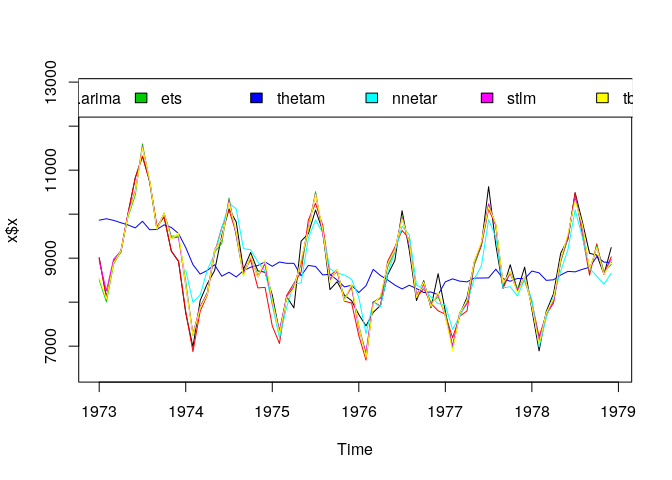``plot(quickModel, type = "models")``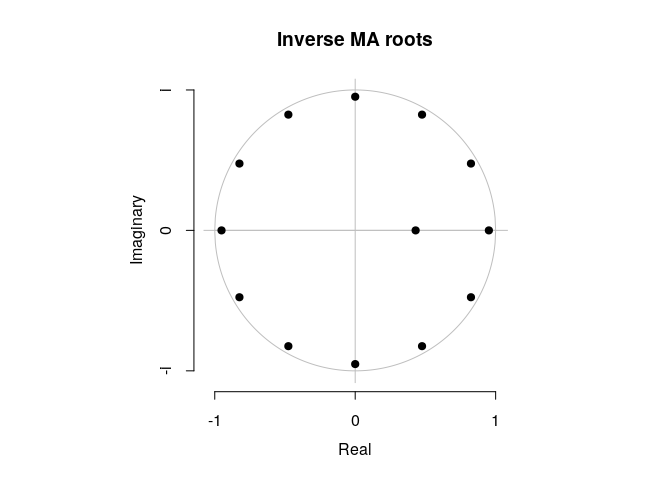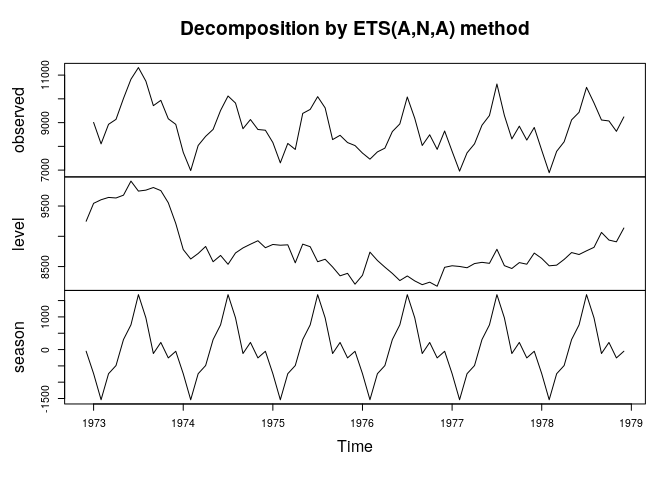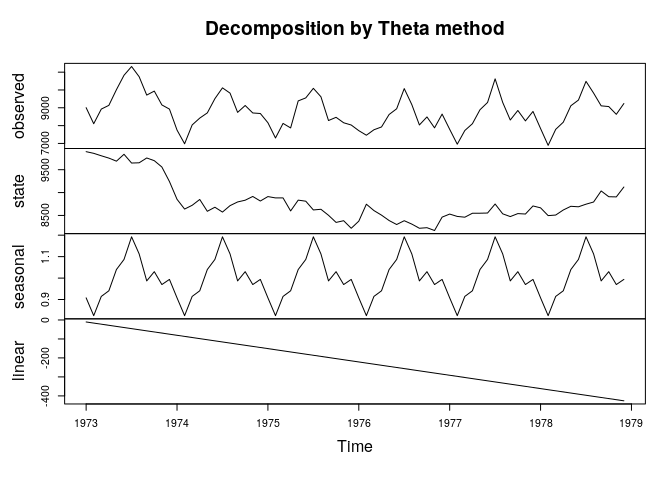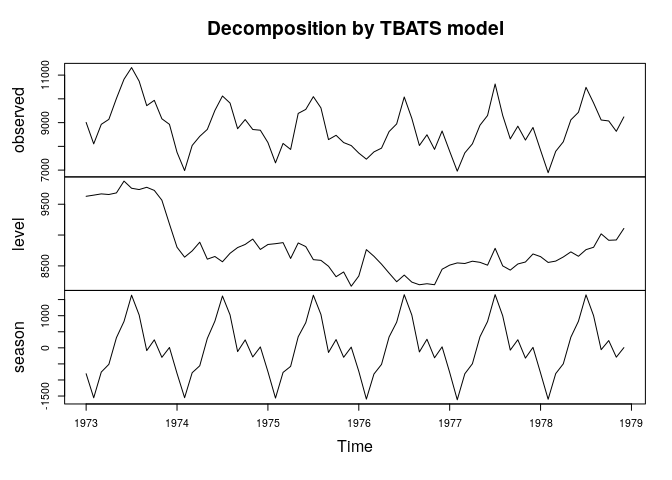Since version 0.4.0, `ggplot` graphs are available. Note, however, that the `nnetar`, and `tbats` models do not have `ggplot::autoplot()` methods, so these are not plotted.

``plot(quickModel, type = "fit", ggplot = TRUE)``
``## Warning: Removed 12 row(s) containing missing values (geom_path).``